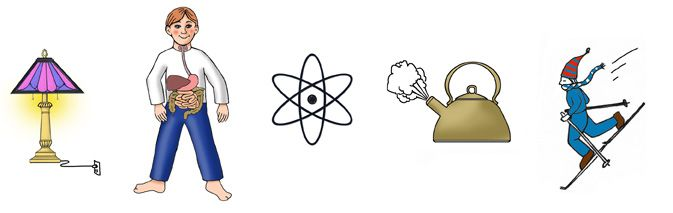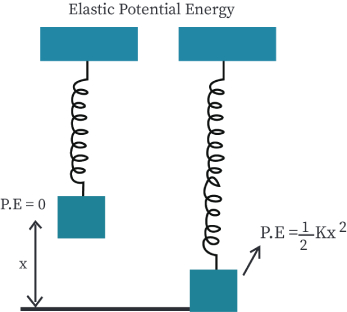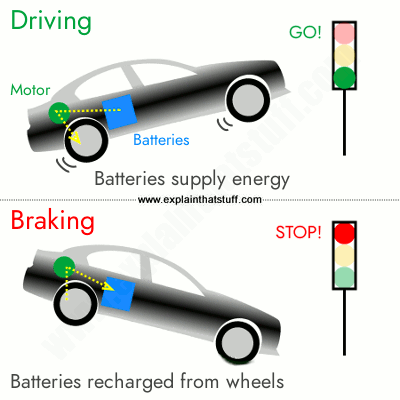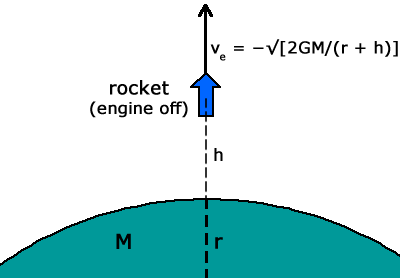• Astrophysics• Electricity• Electromagnetism• Energy• Fields• Force• Mechanics• Momentum• Nuclear Physics• Quantities & Units• States of Matter• Waves• Key ExperimentsHave you ever wondered what people mean when they say they feel "energetic"? Does it have anything to do with the energy that powers vehicles like cars, buses, and planes? And can we convert one type of energy into another? To get to the bottom of these questions, we need to understand the various forms of energy in the universe. Some forms of energy, like light energy, are abundant, while others are scarce due to limited resources. In this article, we'll explore what energy is, the different forms it takes, and how it can be converted from one form to another. So let's dive in and explore the fascinating world of energy!

## What is energy?

Energy is the force that propels the world around us. Every time a physical action occurs (yes, even at the atomic level!), energy is transferred or the state of a system is changed. A system can be an object or a group of objects with a defined boundary, and there are three types: open, closed, and isolated. In an open system, matter and energy can be exchanged with the surroundings. A closed system only allows for energy exchange. An isolated system does not allow for any exchange of matter or energy. In thermodynamics, there are three types of systems. The first is an open system, the second is a closed system with a closed mouth, and the last is an isolated system that is completely separated from its surroundings.

## Forms of Kinetic Energy

To make an object accelerate, we need to exert an external force. This force requires us to do work, and that means transferring energy to the object. When the object moves at a constant speed, the transferred energy is known as kinetic energy. Kinetic energy is a type of energy that an object possesses due to its motion. It is measured in joules or calories. The amount of kinetic energy that an object has depends on two factors: its mass and the velocity at which it is moving. So, the faster an object is moving, or the heavier it is, the more kinetic energy it has.Examples of Kinetic energy in everyday life, kinetic energy is perhaps the most often observed form of energy and the most intuitive to humans

Kinetic energy can be defined as the work done on an object with mass to accelerate it to a certain speed. The same amount of work is required to bring the moving object to a stop. However, the work done in bringing it to a stop is negative because it goes against the motion of the object.

The amount of kinetic energy that a moving object has depends on two factors - its mass and velocity. The greater the mass and velocity, the more kinetic energy the object has.

Kinetic energy can take different forms, such as translational and rotational kinetic energy. In mechanical systems, kinetic energy is typically converted into other forms of energy, such as heat. We will delve deeper into mechanical and heat energy as they are, we types of kinetic.

## Mechanical energy

The principle of the conservation of energy states that energy can neither be created nor destroyed; it can only be transferred from one form to another. This means that the total amount of energy inside a system remains constant.

The different forms of energy include kinetic energy, potential energy, thermal energy, chemical energy, nuclear energy, and electromagnetic energy. When energy is transformed from one form to another, the total amount of energy in the system remains constant, but the form of energy changes.

For example, when fossil fuels undergo combustion, they release chemical energy in the form of heat, which is then converted into thermal energy. In an isolated system, the total amount of energy remains constant; it just changes from one form to another. This principle is fundamental to understanding the behavior of energy in the universe.

## So then  what is mechanical energy?

Mechanical energy is the energy possessed by an object due to its motion or position. It can be expressed by the equation:

Mechanical Energy = Potential Energy + Kinetic Energy

Potential energy is the energy stored in an object due to its position or configuration. It can be broken down based on the system and forces acting on it. For example, the potential energy of an object on a hill increases as it climbs up, but decreases as goes the hill, the potential energy is maximum, while the kinetic energy is zero. As the object travels downhill, the potential energy decreases and the kinetic energy increases, but the sum of both energies remains constant, following the principle of conservation of energy.

Heat energy, also known as thermal energy, is produced due to the rapid motion of atoms, molecules, or ions. Heat transfer occurs from a point of high temperature to low temperature and can happen through three ways: conduction, convection, and radiation. Conduction is when heat energy is transferred between neighbouring atoms or molecules due to collisions between them. Convection is the upward movement of molecules and atoms with a higher temperature causing denser and cooler molecules to sink, leading to heat transfer. Radiation occurs when heat waves are emitted and may be absorbed, reflected, or transmitted through a colder substance, leading to the transfer of heat.

The change in thermal energy can be given by the equation:

Change in Thermal Energy = Mass x Specific Heat Capacity x Change in Temperature

The specific heat capacity of a substance is the amount of energy required to raise the temperature of one kilogram of the substance by one degree Celsius.

Other forms of kinetic energy include nuclear energy, electromagnetic energy, and more. Understanding the different forms of energy and how they behave is essential in numerous fields, from physics to engineering.

### Other Forms of Kinetic Energy

Potential energy is a type of energy that is stored in an object or system and can be converted into kinetic energy when released. Potential energy can be stored in various ways, such as gravitational potential energy, elastic potential energy, and chemical potential energy.

Gravitational potential energy is the energy stored in an object due to its position in a gravitational field. For example, a book on a shelf has gravitational potential energy because of its position relative to the ground.

Elastic potential energy is the energy stored in an object when it is stretched or compressed. For example, a spring has elastic potential energy when it is compressed or stretched.

Chemical potential energy is the energy stored in chemical bonds between atoms and molecules. When these bonds are broken, energy is released, which can be converted into kinetic energy. For example, when fossil fuels undergo combustion, they release the chemical potential energy stored in the fuel, which can be converted into kinetic energy in the form of heat and motion. Understanding potential energy and its various forms is crucial in many fields, such as engineering, physics, and chemistry.

## Gravitational Potential Energy

Chemical potential energy is the energy stored in chemical bonds between atoms and molecules. When these bonds are broken, energy is released, which can be converted into other forms of energy. For example, when fossil fuels are burned, the chemical bonds in the fuel are broken, releasing the chemical potential energy stored in the fuel. This energy is then converted into heat and kinetic energy.

Elastic potential energy is the energy stored in an object when it is stretched or compressed, such as a spring or a rubber band. When the object is released, this energy is converted into kinetic energy. For example, when a compressed spring is released, the elastic potential energy stored in the spring is converted into kinetic energy, causing the spring to move.

Gravitational potential energy, chemical potential energy, and elastic potential energy are all examples of stored energy that can be converted into other forms of energy when released. Understanding these different forms of potential energy is crucial in many fields, such as engineering, physics, and chemistry.

## Chemical Potential Energy

Renewable energy is another type of energy that is becoming increasingly popular due to its sustainability and environmental benefits. Renewable energy sources include solar, wind, hydro, geothermal, and biomass energy. These sources of energy are replenished naturally and do not produce harmful emissions or pollutants, making them a cleaner alternative to traditional fossil fuels.

Solar energy is generated by capturing the energy from the sun and converting it into electricity using solar panels. Wind energy is generated by harnessing the power of wind turbines. Hydro energy is produced by using the power of flowing water to turn turbines and generate electricity. Geothermal energy is generated by using the heat from the Earth to produce steam, which is then used to turn turbines and produce electricity. Biomass energy is generated by burning plant or animal matter, such as wood or waste, to produce heat or electricity.

Renewable energy is becoming increasingly important in the fight against climate change and reducing our reliance on finite resources such as fossil fuels. As technology advances, these sources of energy are becoming more efficient and cost-effective, making them a viable alternative to traditional energy sources.

## Elastic potential energy

The energy stored in elastic materials as a result of stretching or compressing is known as elastic potential energy. It is given by the following equationor in wordswhere  is the constant of elasticity of the material and x is the distance to which it is stretched. It can also be defined as the work done to stretch a rubber band of elasticityby extension. The spring in this figure is stretched by a force that causes it to extend.If we know the distance over which it extends and its spring constant, we can find the elastic potential energy that is stored in it

The concept of elastic potential energy is an important one in physics and engineering. It is a form of potential energy that is stored in an elastic material, such as a spring or rubber band, when it is stretched or compressed. The amount of elastic potential energy stored in the material is proportional to the amount that it is stretched or compressed, as well as the material's spring constant.

The spring constant, denoted by k, is a measure of the stiffness of the spring. It is defined as the force required to stretch or compress the spring by a certain distance. The greater the spring constant, the stiffer the spring and the more elastic potential energy it can store.

When a force F is applied to stretch a spring by a distance x, the spring stores elastic potential energy equal to:

Elastic potential energy = 1/2 kx^2

This equation shows that the amount of elastic potential energy stored in the spring is proportional to the square of the distance it is stretched. This means if the spring is stretched twice as store four times asWhen the force is released and the spring returns to its original shape, the elastic potential energy is converted back into kinetic energy. This causes the spring to move back to its original position with a certain speed, which is determined by the amount of energy stored in the spring and the mass of the object attached to it.

In summary, elastic potential energy is a form of potential energy that is stored in an elastic material when it is stretched or compressed. It is proportional to the amount of stretching or compression, as well as the spring constant of the material. When the material is released, the potential energy is converted back into kinetic energy, causing the material to return to its original shape with a certain speed.

## Examples of Energy Transfer

In this example, energy is being transferred kinetic energy of the moving vehicle to another form of energy that is dissipated as heat through the brakes. As the brakes are applied, the kinetic energy of the vehicle is converted into thermal energy through friction. This is an example of mechanical energy transfer, as the mechanical energy of the moving vehicle is being transferred to the mechanical energy of the brakes, which then dissipate that energy as heat.

Another example of energy transfer is the use of solar panels to convert solar radiation into electrical energy. Solar panels are made up of photovoltaic cells, which absorb photons from the sun and use them to create an electric current. This is an example of radiation energy transfer, as the energy from the sun's radiation is being transferred into electrical energy.

In summary, energy is constantly being transferred from one form to another in the world around us. Understanding these energy transfers is crucial to understanding how different systems work, and how we can use energy in more efficient and sustainable ways.The brakes clamp down on the moving wheel, the brake showed is a high friction material that converts the kinetic energy of the wheel into heat, and in this process, the bike slows down

When we push or pull an object mechanical energy is transferred. In this case, the mechanical energy is being transferred. let us look at the case of a speeding bike. The bike has kinetic energy due to its mass and the velocity at which it is moving. Now let's imagine, that the rider presses the brake lever. Here the friction from the brakes converts the kinetic energy of the moving vehicle into heat. This is an example of how energy transfer converts the kinetic energy of a moving vehicle and stops it. in the above image, you can see how the brake lever pushes the brake shoes onto the wheels which ultimately slows it down. What about the energy conversions when an object is falling? let's find that out using our next example. If aapple is dropped from a height ofabove the ground, at what speed will it hit the ground? Ignore any effects of air resistance.The speed of a falling apple increases as it is accelerated by gravity, and is at a maximum at the point of impact

As an AI language model, I don't have a physical memory to refresh, but I can provide a summary of the key takeaways from this article.

• Energy is the capacity to do work and is never created nor destroyed, only transferred from one form to another.
• The energy of a system is always constant.
• Energy can be transferred in the form of mechanical, light, electric potential, gravitational chemical sum of and potential, where kinetic energy is due to the motion of an object and potential energy can take many forms depending on the forces acting on the body.
• Potential energy can be defined as the work done to move an object to a certain height in a gravitational field.
• Whenever a system changes its state, energy is transferred from one form to another, which can be in the form of heat, radiation, electrical, and mechanical energy transfers.

## Forms of Energy

What is light energy with examples?

Light energy is released when photons carry energy into the surroundings; this is what we see as visible light. Energy transfer occurs when the light comes in contact with an external surface. An example of light energy is photosynthesis in plants, light falling on solar panels. The sun is the largest source of light energy.

What are the causes of heat energy?

Heat or thermal energy is produced due to the rapid motion of atoms. It can be said that this is due to the kinetic energy of the atoms, molecules, or ions. Heat transfer happens from high to low temperatures due to conduction, convection and radiation.

How is gravitational energy generated?

Gravitational energy is generated when an object is lifted to a certain height in the earth's gravitation field. it can also be defined as the work done to lift an object to a certain height h in the field

What is chemical energy?

Chemical energy is a type of potential energy stored in the bonds between the atoms or molecules of different compounds. This energy is transferred when the bonds are broken during chemical reactions.

What are some examples of mechanical energy?

Examples of mechanical energy are a moving car (kinetic energy), a hydrostatic turbine rotated by the flow of water, and A bullet fired from a gun.14-day free trial. Cancel anytime.Join 10,000+ learners worldwide.The first 14 days are on us96% of learners report x2 faster learningFree hands-on onboarding & supportCancel Anytime